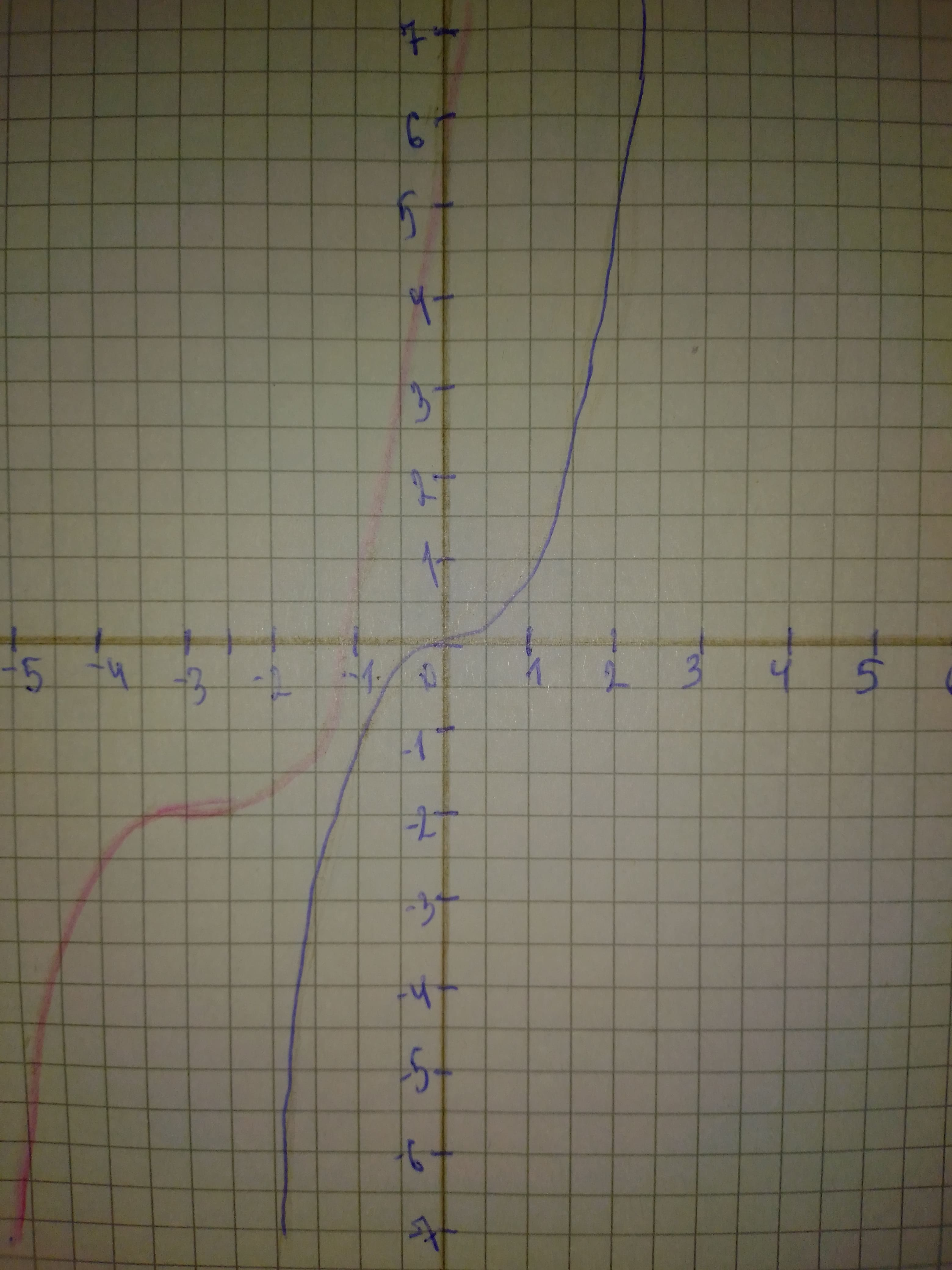# a) Identify the parameters a, b, h, and k in the polynomial y=frac{1}{3}(x+3)^{3}-2 Describe how each parameter transforms the base function y=x^{3}.ddaeeric 2021-02-25 Answered
a) Identify the parameters a, b, h, and k in the polynomial $y=\frac{1}{3}{\left(x+3\right)}^{3}-2$ Describe how each parameter transforms the base function $y={x}^{3}$.
b) State the domain and range of the transformed function.
c) Sketch graphs of the base function and the transformed function on the same set of axes.
You can still ask an expert for help

• Questions are typically answered in as fast as 30 minutes

Solve your problem for the price of one coffee

• Math expert for every subject
• Pay only if we can solve itLaaibah Pitt

Step 1
Work as shown below, follow the steps:
a) Use the general transformation equation: $y=af\left(b\left(x-h\right)\right)+k$
We are given the transformed function: $y=\frac{1}{3}{\left(x+3\right)}^{3}-2$
By finding the values of the parameters a,b,c,d we see that the graph of the parent function $y={x}^{3}$ will undergo the following transformations:
-No horizontal stretch, because $b=1$
-A vertical stretch by a factor of $\frac{1}{3}$ because $a=\frac{1}{3}$
-A horizontal translation 3 units left because $h=-3<0$
-A vertical translation 2 units down because $k=-2<0$
Step 2
For the domain:
The end behavior for this function is Down on the left and Up on the right, so this function can get all values in the set of real numbers (vertical axis) so:
The range is: $R=\left\{y\mid y\in R\right\}$
Step 3
For the graph of the given transformed function start from the graph of the parent function $y={x}^{3}$
Apply the transformations from part a) to the graph of $y={x}^{3}$ to obtain the graph of the transformed function $y=\frac{1}{3}{\left(x+3\right)}^{3}-2$
See in the following picture: